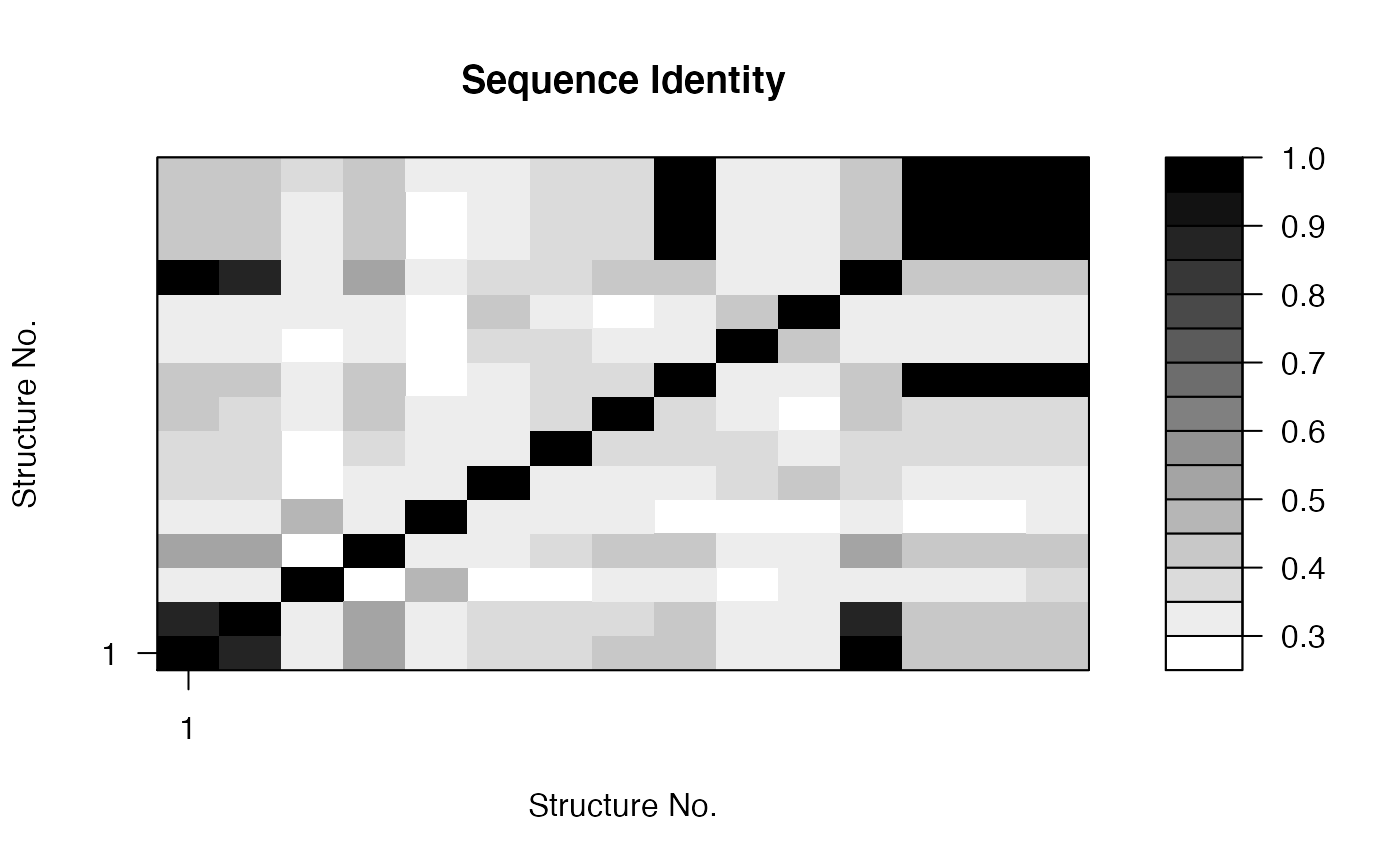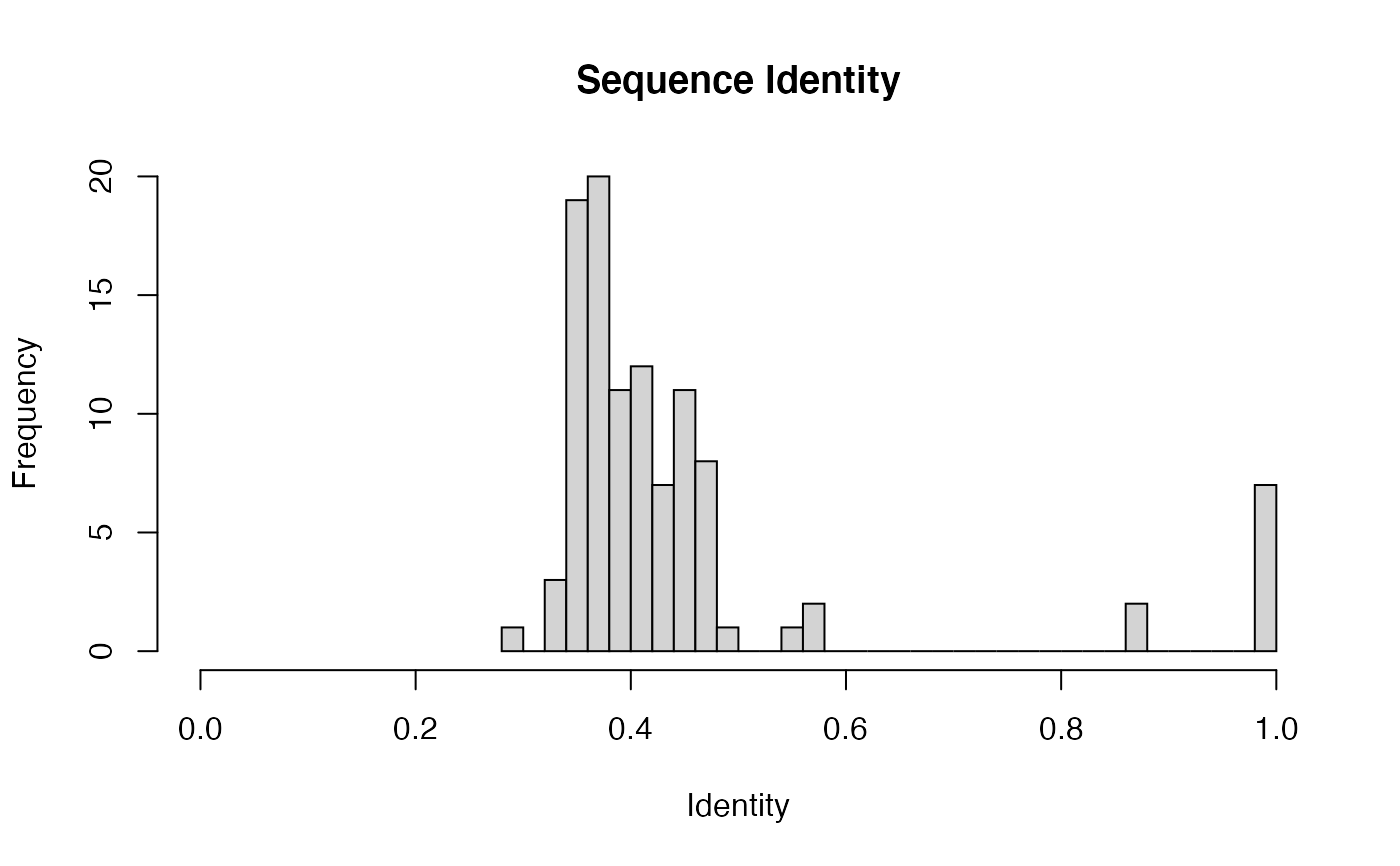Determine the percent identity scores for aligned sequences.

seqidentity(alignment, normalize=TRUE, similarity=FALSE, ncore=1, nseg.scale=1)

## Arguments

alignment sequence alignment obtained from read.fasta or an alignment character matrix. logical, if TRUE output is normalized to values between 0 and 1 otherwise percent identity is returned. logical, if TRUE sequence similarity is calculated instead of identity. number of CPU cores used to do the calculation. ncore>1 requires package ‘parallel’ installed. split input data into specified number of segments prior to running multiple core calculation. See fit.xyz.

## Details

The percent identity value is a single numeric score determined for each pair of aligned sequences. It measures the number of identical residues (“matches”) in relation to the length of the alignment.

## Value

Returns a numeric matrix with all pairwise identity values.

Grant, B.J. et al. (2006) Bioinformatics 22, 2695--2696.

## Author

Barry Grant

read.fasta, filter.identity, entropy, consensus

## Examples


attach(kinesin)

ide.mat <- seqidentity(pdbs)

# Plot identity matrix
plot.dmat(ide.mat, color.palette=mono.colors,
main="Sequence Identity", xlab="Structure No.",
ylab="Structure No.")# Histogram of pairwise identity values
hist(ide.mat[upper.tri(ide.mat)], breaks=30,xlim=c(0,1),
main="Sequence Identity", xlab="Identity")# Compare two sequences
seqidentity( rbind(pdbs$ali[1,], pdbs$ali[15,]) )
#>       [,1]  [,2]
#> [1,] 1.000 0.461
#> [2,] 0.461 1.000
detach(kinesin)